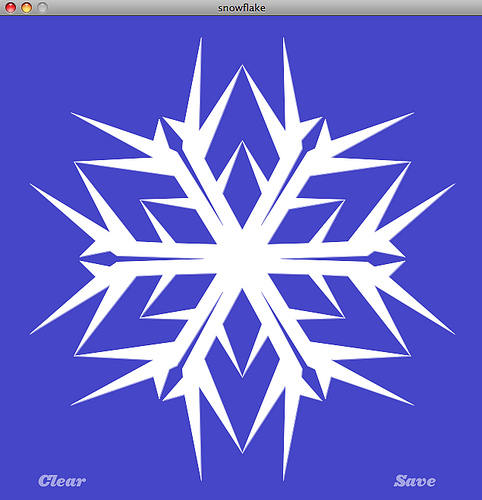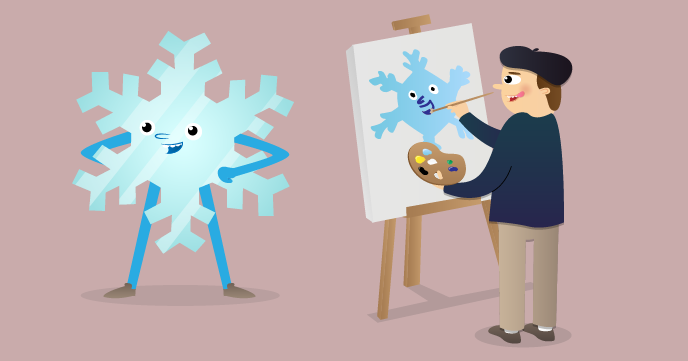# Kaleidoscopes, rotation, reflection and colour fills.## Symmetries

For two-dimensional figures, rotational symmetry governs how many times a pattern repeats round 360 degrees, while adding reflections mirrors the objects within their sector.

LB has the easy ability to call Windows dll's, in particular one to read a pixel's colour, and another to fill to the boundary of a region. Together these make for a lot of colourful fun!

The code below allows you to choose what symmetry to use, and draws the black outline. Be careful to draw slowly and leave no gaps. You can then choose a colour for each area, and the corresponding areas all will be coloured too. A further option is to get it to flash each area independently of its symmetry partners in random colours. You have too an option to save-as=bitmap.

By calling ImageMagick you could save as GIF or create animations. I find it easy to do these 'by hand'.

Output of other related versions- you can add random colour fills within the LB code, or add them with an area-fill tool in your favourite image processing software;--Note this is still a work-in-progress. Plenty of ideas still for development, see the remmed lines for examples!

```

'**********************************************************************
'**                                                                  **
'**      kaleidoscope9.bas    tenochtitlanuk  June 2018              **
'**                                                                  **
'**********************************************************************

' to-dos:
'     fast movement leaves gaps. Save all previous positions and 'echos'?
'     add an 'undo' to the fill?      NO Just re-use 'fill'.
'     change 'save' routine so won't  save over another saved image.          DONE
'     check area allowed to draw in and show appropriately ( as originally).  DONE
'     Guidance notes.                                                         DONE
'     Undo the arc before saving. ( overwrite in background colour?           DONE

global radius, theta, hdc, hw, targetcolor, pi
pi    =4 *atn( 1)

nomainwin ' un-rem for debugging..

BackgroundColor\$ = "red"

WindowWidth  =900
WindowHeight =600

button #w.b1, "Save Image", [saveImage], LR, 110, 20
button #w.b2, "Sparkle",    [sparkle],   LR, 110, 80

statictext #w.st,  "", 610,  30, 260, 280
statictext #w.st2, "", 650, 330, 170,  40

notice "Edit values of N and kal to alter symmetries used."
' _________________________________________________________________________________________________

N     =5                                          '   number of rotations /symmetry   <<<<<<<<<<

kal   =0    '   kal =0 for N-fold symmetry, kal =1 for added kaleidoscope reflections.<<<<<<<<<<
' ________________________________________________________________________________________________

open "Paint your star!" for graphics_nsb as #w

s\$  ="" +chr\$( 13)+_
" Hold down LeftButton and drag in" +chr\$( 13) +_
"   marked zone to draw." +chr\$( 13) +chr\$( 13)+_
" Right click to get a colour selector" +chr\$( 13) +_
"   to fill am area." +chr\$( 13) +chr\$( 13)+_
"    NB You can ONLY draw in the" +chr\$( 13) +_
"    indicated area. Rotations and" +chr\$( 13) +_
"    mirroring apply automatically."  +chr\$( 13) +chr\$( 13)+_
"    Edit N and kal to alter symmetry &" +chr\$( 13) +_
"    rotations and whether you want" +chr\$( 13) +_
"    Kaleidoscope reflections." +chr\$( 13) +chr\$( 13)+_
" Save button allows your creation" +chr\$( 13) +_
"   to be saved."

hw =hwnd( #w)                                          '    <<<<<<<<<<<<<<<<<
calldll #user32, "GetDC", hw as ulong, hdc as ulong    '    <<<<<<<<<<<<<<<<<

#w "trapclose quit"

#w "when leftButtonMove  [paint]"
#w "when rightButtonUp   [colFill]"

#w "color 240 240 240 ; flush"

#w.st s\$
#w.st2 " Current rotations ="; N
if kal =0 then
#w.st2 " Current rotations ="; N; " Reflections are OFF."
else
#w.st2 " Current rotations ="; N; chr\$( 13); " Reflections are ON."
end if

th1   =int( 360 /N /2)
#w "color black"

if kal =0 then
call pie 300, 300,   252,     0 -th1, 2 *th1
else
call pie 300, 300,   252,     0,         th1
end if

#w "backcolor 240 240 240"
#w "up ; size 3 ; goto 300 300 ; down ; circle 250 ; size 4"    '   large size so gaps less likely
#w "color black"

wait

[paint]
x       =MouseX -300
y       =300 -MouseY
theta   =180 /pi *atan2( y, x)
if theta >180 /N or radius <20 or radius >250 then wait

if kal =1 then
if theta <0 -180 /N or theta >=0      then wait
else
if theta <0 -180 /N or theta >=180 /N then wait
end if

for i =0 to N -1
angle   =i *360 /N
if kal =1 then call set radius,   angle +theta
next i

wait

[colFill]
xVar   =MouseX -300:  yVar   =MouseY -300   '   w.r.t. centre of graphic area
print "Mouse clicked for fill colour at "; xVar; ", "; yVar; " from centre."
colordialog "0 0 0",    fillCol\$
if fillCol\$ ="" or fillCol\$ ="black" or fillCol\$ ="0 0 0" then [colFill]

#w "color "; fillCol\$; " ; backcolor "; fillCol\$

targetcolor     =0  '   this is the colour of the outline to fill out to.

theta   =180 /pi *atan2( yVar, xVar)

print " This was radius "; int( radius); " angle "; int( theta); " degrees clockwise w.r.t. centre."

for i =0 to N -1
angle   =i *360 /N
xV      =300 +int( radius *cosRad( angle +theta))  '   using coordinates w.r.t. top left as origin
#w "size 8 ; down ; set "; xV; " "; yV
#w "up ; size 3"
print "  Fill from "; using( "####", xV); " "; using( "####", yV)

calldll #gdi32, "ExtFloodFill",_
hdc                as ulong,_
xV                 as long,_
yV                 as long,_
targetcolor        as long,_
_FLOODFILLBORDER   as long,_    'ie fill out 'til this colour is met... <<<<<<<<<<<<<<<<<<<<<<<<<<
result             as long

print "  Fill from "; using( "####", xV); " "; using( "####", yV)

calldll #gdi32, "ExtFloodFill",_
hdc                as ulong,_
xV                 as long,_
yV                 as long,_
targetcolor        as long,_
_FLOODFILLBORDER   as long,_    'ie fill out 'til this colour is met... <<<<<<<<<<<<<<<<<<<<<<<<<<
result             as long

next i

#w "color black ; down"
scan
wait

end function

end function

[saveImage]
#w "color 180 180 40; size 4 ; down"

if kal =0 then
call pie 300, 300,   252,     0 -th1, 2 *th1
else
call pie 300, 300,   252,     0,         th1
end if

#w "getbmp scr 0 0 595 580"
filedialog "Save As...", "default.bmp", fn\$
if fn\$ <>"" then bmpsave "scr", fn\$ else bmpsave "scr", "default.bmp"

#w "color black"
wait

sub set r, t
if r <250 then
t =t *pi /180
x =300 +r *cos( t): y =300 +r *sin( t)
#w "set "; x; " "; y
end if
end sub

sub pie x, y,    r,     startAngle, spanAngle
#w "down ; size 2"

for i =startAngle to startAngle +spanAngle
xs =int( 1.04 *r *cosRad( i))
ys =int( 1.04 *r *sinRad( i))
#w "set "; 300 +xs; " "; 300 +ys
next i
end sub

function atan2( y, x)
pi =atn( 1) *4
if x <>0 then arctan = atn( y /x)
select case
case x >0
atan2 =arctan
case y >=0 and x <0
atan2 =pi +arctan
case y <0 and x <0
atan2 =arctan -pi
case y >0 and x =0
atan2 =pi /2
case y <0 and x =0
atan2 =pi /-2
end select
end function

sub quit h\$
calldll #user32, "ReleaseDC", hw as ulong, hdc as ulong, ret as void   'release the DC    <<<<<<
close #w
end
end sub

[sparkle]
for sp =1 to 2000
[pt]
x =int( 500 *rnd( 1)): y =int( 500 *rnd( 1))
if ( ( x -250)^2 +( y -250)^2)^0.5 >=248 then [pt]
xF =x +50
yF =y +50

calldll #gdi32, "GetPixel", hdc as ulong, x as long, y as long,      pixcol as long
bl = int(  pixcol /( 256*256))
gr = int( (pixcol               -bl *256*256) / 256)
re = int(  pixcol               -bl *256*256 - gr *256)

if pixcol <>0 then
r\$      =str\$( 56 +int( 200 *rnd( 1)))
g\$      =str\$( 56 +int( 200 *rnd( 1)))
b\$      =str\$( 56 +int( 200 *rnd( 1)))
choice\$ =r\$ +" " +g\$ +" " +b\$

#w "backcolor "; choice\$

calldll #gdi32, "ExtFloodFill",_
hdc                as ulong,_
xF                 as long,_
yF                 as long,_
targetcolor        as long,_
_FLOODFILLBORDER   as long,_    '   '   ie fill out 'til this colour is met... <<<<<<<<<<<<<<<<<<<<<<<<<<
result             as long

scan
'calldll #kernel32, "Sleep", 50 as long, ret as void
end if

next sp
wait

'sub q h\$
'  close #wM
'  end
'end sub

```